Courses

# Electrical Machines - MCQ Test 2

## 20 Questions MCQ Test GATE Electrical Engineering (EE) 2022 Mock Test Series | Electrical Machines - MCQ Test 2

Description
This mock test of Electrical Machines - MCQ Test 2 for GATE helps you for every GATE entrance exam. This contains 20 Multiple Choice Questions for GATE Electrical Machines - MCQ Test 2 (mcq) to study with solutions a complete question bank. The solved questions answers in this Electrical Machines - MCQ Test 2 quiz give you a good mix of easy questions and tough questions. GATE students definitely take this Electrical Machines - MCQ Test 2 exercise for a better result in the exam. You can find other Electrical Machines - MCQ Test 2 extra questions, long questions & short questions for GATE on EduRev as well by searching above.
QUESTION: 1

### The starting current of a 3ϕ induction motor is 5 times the rated current, while the rated slip is 4%. The ratio of starting torque to full-load torque is

Solution: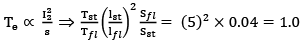QUESTION: 2

### A single-phase transformer when supplied from 220V, 50 Hz has eddy current loss of 50W. If the transformer is connected to a voltage of 330V, 50 Hz, the eddy current loss will be

Solution: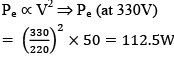QUESTION: 3

### If Pc and Psc represent core and full-load ohmic losses respectively, the maximum kVA delivered to load corresponding to maximum efficiency is equal to rated kVA multiplied by

Solution:
QUESTION: 4

A d.c. series motor is accidently connected to single-phase a.c. supply. The torque produced will be

Solution:

In dc series motor, the ac currents through the field & armature windings will always be in the same direction. So torque will be unidirectional but pulsating due to ac.

QUESTION: 5

The ‘synchronous-impedance method’ of finding the voltage regulation by a cylindrical rotor alternator is generally considered as

Solution:

As unsaturated value of Zs is more than the saturated value, voltage regulation computed by emf method is much higher than the actual value. It is because of this reason that the emf method is called a pessimistic method.

QUESTION: 6

Generally the no-load losses of an electrical machine is represented in its equivalent circuit by a

Solution:

No-load losses (essentially core losses not F & W losses) are low and depend upon the applied voltage in an induction machine.

QUESTION: 7

The power factor of a synchronous motor

Solution:

Refer, inverted V-curves related to V-curves.

QUESTION: 8

A 6-pole, 3-phase alternator running at 1000 rpm supplies to a 8-pole, 3-phase induction motor which has a rotor current of frequency 2 Hz. The speed at which the motor operates is

Solution: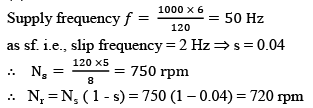QUESTION: 9

For maximum current during ‘Slip Test’ on a synchronous machine, the armature mmf aligns along

Solution:
QUESTION: 10

A 3-phase 50 MVA 10kV generator has a reactance of 0.2 ohm per phase. Hence the per-unit value of the reactance on a base of 100 MVA 25 kV will be

Solution:
QUESTION: 11

The results of a ‘Slip-Test’ for determining direct-axis (Xd) and quadrature-axis (Xq) reactances of a star-connected, salient-pole alternator are given below:

Phase values: Vmax = 108V; Vmin = 96V, Imax = 12A, Imin = 10A. Hence the two reactances will be

Solution: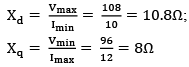QUESTION: 12

A two-winding transformer is used as an auto-transformer. The kVA rating of the auto-transformer compared to the twowinding transformer will be

Solution:

Answer would depend upon the voltage ratio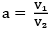and type of auto-transformer
i.e.., set-up or step-down.
For step-up transformer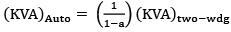Where a < 1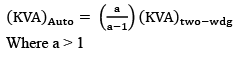Let, N1 = N2 (Ideal two winding transformer)   For step-up transformer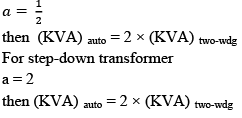QUESTION: 13

A 20 kVA, 2000/200V, 1-phase transformer has name-plate leakage impedance of 8%. Voltage required to be applied on the high-voltage side to circulate full-load current with the low-voltage winding short-circuited will be

Solution:

V = 0.08 × 1 pu
∴ V actual = 0.08 × 2000 = 160V

QUESTION: 14

The full-load copper-loss and iron loss of a transformer are 6400W and 5000W respectively. The copper-loss and ironloss at half load will be, respectively

Solution:

Copper loss = (1/2)2 × 6400 = 1600W Iron loss does not depend upon the load but upon the applied voltage.

QUESTION: 15

In a 100 kVA, 1100/220V, 50 Hz single-phase transformer with 2000 turns on the high-voltage side, the open-circuit test result gives 200V, 91 A, 5kW on low-voltage side. The coreloss component of current is approximately

Solution: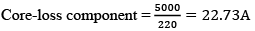QUESTION: 16

For a given torque, reducing the diverter-resistance of a d.c. series motor

Solution: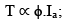reducing the diverter-resistance reduces the ϕ, so for a given load torque, Ia would increase.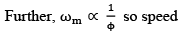would also increase.

QUESTION: 17

Possible three-to-three phase transformer connection for parallel operation is

Solution:

To ensure co-phasor primary and secondary windings.

QUESTION: 18

A 4 kVA, 400/200V single-phase transformer has resistance of 0.02 p.u. and reactance of 0.06 p.u. Its actual resistance and reactance referred to high voltage side are respectively

Solution: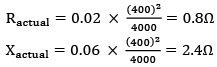QUESTION: 19

Two 10 kV/440V, 1-phase transformers of ratings 600 kVA and 350 kVA are connected in parallel to share a load of 800 kVA. The reactances of the transformers, referred to the secondary side are 0.0198Ω and 0.0304Ω respectively (resistances negligible). The load shared by the two transformers will be respectively

Solution: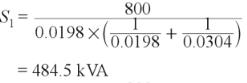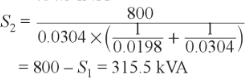QUESTION: 20

The current drawn by a 120V d.c. motor with back e.m.f. of 110V and armature resistance of 0.4 ohm is

Solution:

Eb = Vt – Ia Ra
∴ 110 = 120 – Ia × 0.4   ⇒ Ia = 25A